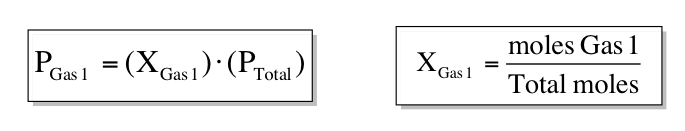Ch.1 - Intro to General Chemistry 2hrs & 53mins 0% complete Worksheet Ch.2 - Atoms & Elements 2hrs & 49mins 0% complete Worksheet Ch.3 - Chemical Reactions 3hrs & 25mins 0% complete Worksheet BONUS: Lab Techniques and Procedures 1hr & 38mins 0% complete Worksheet BONUS: Mathematical Operations and Functions 47mins 0% complete Worksheet Ch.4 - Chemical Quantities & Aqueous Reactions 3hrs & 30mins 0% complete Worksheet Ch.5 - Gases 3hrs & 47mins 0% complete Worksheet Ch.6 - Thermochemistry 2hrs & 28mins 0% complete Worksheet Ch.7 - Quantum Mechanics 2hrs & 35mins 0% complete Worksheet Ch.8 - Periodic Properties of the Elements 1hr & 57mins 0% complete Worksheet Ch.9 - Bonding & Molecular Structure 2hrs & 5mins 0% complete Worksheet Ch.10 - Molecular Shapes & Valence Bond Theory 1hr & 31mins 0% complete Worksheet Ch.11 - Liquids, Solids & Intermolecular Forces 3hrs & 40mins 0% complete Worksheet Ch.12 - Solutions 2hrs & 17mins 0% complete Worksheet Ch.13 - Chemical Kinetics 2hrs & 22mins 0% complete Worksheet Ch.14 - Chemical Equilibrium 2hrs & 26mins 0% complete Worksheet Ch.15 - Acid and Base Equilibrium 4hrs & 42mins 0% complete Worksheet Ch.16 - Aqueous Equilibrium 3hrs & 48mins 0% complete Worksheet Ch. 17 - Chemical Thermodynamics 1hr & 44mins 0% complete Worksheet Ch.18 - Electrochemistry 2hrs & 58mins 0% complete Worksheet Ch.19 - Nuclear Chemistry 1hr & 33mins 0% complete Worksheet Ch.20 - Organic Chemistry 3hrs 0% complete Worksheet Ch.22 - Chemistry of the Nonmetals 2hrs & 1min 0% complete Worksheet Ch.23 - Transition Metals and Coordination Compounds 1hr & 54mins 0% complete Worksheet

# Partial Pressure

See all sections

According to Dalton’s Law, the total pressure inside a container is obtained by adding all the partial pressures of each non-reacting gas.

###### Examining Dalton's Law

Concept #1: Understanding Dalton's Law

Transcript

Welcome back guys. In this video, we're going to take a look at the partial pressure of gases. Now, what do I mean by partial pressure? What we said in the beginning, that pressure equals force over area. We saw a picture of the container with several different types of gas particles. We're going to say that the total pressure inside of that container comes from all the pressures that each of those particles added up.
Let's say we wanted to look at the pressure of one of those gas particles and ignore the others. The pressure of that one single gas particle would be that gas particles partial pressure. It just means the pressure of a gas when it surrounded by a bunch of different gases. We're not looking at the total pressure. We're looking at that gas particles individual pressure. That individual pressure is partial pressure.
We're going to say Dalton's law states that, 'In a container of unreacting gases,' that means that these gases are just bouncing off of each other. They're not connecting together to give me a new compound. 'The total pressure of the container is the sum of the partial pressure of the individual gases.' All these mean is let's say we have a container and its filled with three types of gases. It's filled with O2, argon and neon gas.
Let's say that the pressure of the oxygen gas is 0.3 atm and argon is 0.1 atm and neon is 0.3 atm. We'd say that each of them has those individual pressures—that's their partial pressures. Dalton's law says, while the total pressure inside of the container is just each of them added up together. According to Dalton's law, the total pressure equals 0.7 atm, and that 0.7 atm comes from us adding up each of those individual pressures together.

###### Mole Fraction & Partial Pressure

Concept #2: Understanding mole fraction and partial pressure

Transcript

We're going to say that the total pressure due like we said to the total number of moles of each of these gas particles. So pressure and moles are connected together. We're going to say the partial pressure of each gas molecule is a total pressure multiplied by the mole fraction of each gas particle or gas molecule. What the heck does that mean?
What I'm saying here is, the pressure of a gas, of any individual gas is equal to its mole fraction, mole fraction is X. We're going to say, 'What the heck is mole fraction?' Mole fraction is the moles of that gas divided by the total moles of all the gases. If we're looking for example the mole fraction of neon gas, it'd be the moles of neon divided by the moles of oxygen, argon plus neon on the bottom. That would give us the mole fraction of neon.
Once we get the mole fraction of a gas, we would multiply times the total pressure. That would then give me just the pressure of that one gas. Here this is just partial pressure. I know these ideas are kind of abstract, so that's why we're going to move on to the next question, the example. This is going to show us how exactly does, how do we find the partial pressure of the gas and does that relate to the mole fraction of that gas.

The partial pressure of a gas is based on the moles of that gas. The relationship of the different moles of gases helps us determine the mole fraction of each gas.Example #1: A container has 16.7 g O2, 8.1 g H2 and 35.2 g N2 and contains a total pressure of 0.83 atm. Calculate the mole fraction of O2 and its partial pressure.

Practice: A gas mixture with a total pressure of 812 mmHg contains the following gases at with their partial pressures: Cl= 210 mmHg, H2 = 180 mmHg, CO2 = 215 mmHg. If argon gas is also present calculate its mole fraction.